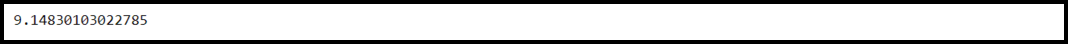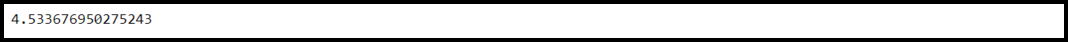Open in App
Not now

# How to Find the F Critical Value in Python?

• Last Updated : 21 Feb, 2022

F-critical value is a particular value that we used to compare our f value to. While conducting the F test we obtain F statistics as an outcome. For determining whether the result of the f test is statistically significant the f statistic is compared with the F critical value. If the F statistic is larger than the F critical value then we assume that the result of the test is statistically significant.

This article focuses upon finding F critical value in Python. Before proceeding further we need Scipy library already installed in our system. You can install this library by using the below command on your terminal,

`pip3 install scipy`

Calculating F critical value in Python is a step by step process,

Step 1: Import the Scipy library.

The very first step is to import the Scipy library. It is used for scientific computation and uses NumPy internally.

## Python3

 `# Importing library ` `import` `scipy.stats`

Step 2: Define parameters.

To calculate F-Critical value we need these parameters: A significance level, Numerator degrees of freedom, Denominator degrees of freedom. As an example, we have assumed respective values,

significance level = 0.01, numerator degrees of freedom = 4, and denominator degrees of freedom = 6

Step 3: Calculate F-Critical value.

For calculating F-Critical value scipy.stats provide us scipy.stats.f.ppf() function using which we can calculate the F-Critical value. The syntax is given below,

Syntax:

scipy.stats.f.ppf(q, dfn, dfd)

Parameters:

q: It represents the significance level to be used

dfn: It represents the numerator degrees of freedom

dfd: It represents the denominator degrees of freedom

Return Type:

Returns the critical value from the F-distribution

Example:

## Python3

 `# Importing library ` `import` `scipy.stats ` ` `  `# Determine the F critical value ` `print``(scipy.stats.f.ppf(q``=``1``-``.``01``, dfn``=``4``, dfd``=``6``)) `

Output:F-Critical value

Hence, The F critical value for a significance level of 0.01, numerator degrees of freedom = 4, and denominator degrees of freedom = 6 is 9.148.

Bonus:

The alpha value is inversely proportional to the critical values. For example, consider the below program having the alpha value = 0.05, numerator degrees of freedom = 4, and denominator degree of freedom = 6 (the latter two are the same as the above-taken example).

Example:

## Python3

 `# Importing library ` `import` `scipy.stats ` ` `  `# Determine the F critical value ` `print``(scipy.stats.f.ppf(q``=``1``-``.``05``, dfn``=``4``, dfd``=``6``)) `

Output:F-Critical value

Hence, The F critical value for a significance level of 0.05, numerator degrees of freedom = 4, and denominator degrees of freedom = 6 is 4.533.

My Personal Notes arrow_drop_up
Related Articles